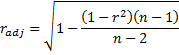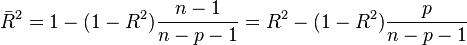For every model of relationship between y and xIf all the observations are on the model then r^2=1

Fortunately r turns out to be normalized covariance    🙂http://maths.nayland.school.nz/Year_13_Maths/3.9_Bivariate_data/7_Coefficient_of_determination.htmlwhere p is the total number of regressors in the linear model (not counting the constant term), and n is the sample size.

Adjusted R2 can also be written as$\bar R^2 = {1-{SS_\text{res}/df_e \over SS_\text{tot}/df_t}}$

where dft is the degrees of freedom n– 1 of the estimate of the population variance of the dependent variable, and dfe is the degrees of freedom np – 1 of the estimate of the underlying population error variance.

The principle behind the adjusted R2 statistic can be seen by rewriting the ordinary R2 as$R^{2} = {1-{\textit{VAR}_\text{err} \over \textit{VAR}_\text{tot}}}$

Since 11 April 2023: 186 total views,  1 views today